# How Solve Any Linear Equation in python

This function can solve any linear equation in three lines of code — it could even be rewritten in two lines. As of my knowledge, this is the most efficient method to solve a linear equation in Python.

``````def solve_linear(equation,var='x'):
expression = equation.replace("=","-(")+")"
grouped = eval(expression.replace(var,'1j'))
return -grouped.real/grouped.imag

solve_linear('x - 6 = 10')
16.0

solve_linear('x*1/2 - 6 = 10')
32.0
``````

## How does it work?

First, let’s define what a linear equation is — it must be solvable in its pure form. This means that it can only have one variable, usually written as x. A two-variable equation would require multiple linear equations (a system of equations) to be solved.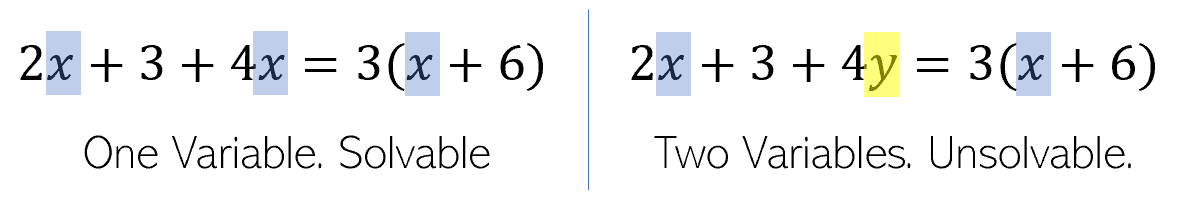python linear equation 1

A linear equation consists of three primary components — constants, variables, and multipliers.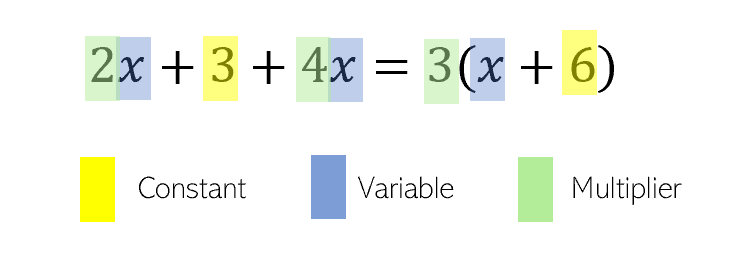python linear equation 2

Any number or combination of operations — addition, subtraction, multiplication, and division — are valid, with any parenthesis scope. As long as it abides by these definitions of a linear equation, it is solvable by our function.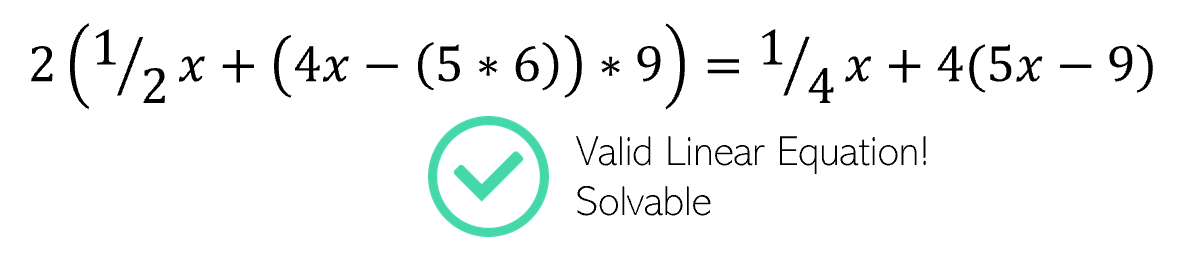python linear equation 3

Let’s break down the function step-by-step. As an example, we will be using the following linear equation as a demonstration.

``````2x + 3 = x - 6
``````

The first line transforms the equation into an expression to be evaluated by moving the entire expression on the right side of the equation to the left side.

``````expression = equation.replace("="," - (")+")"
``````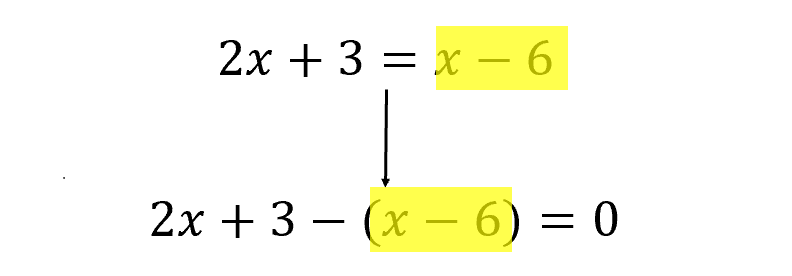python linear equation 4

All the 'information' in the equation has been shifted to one side, and while the equals sign has been discarded, remember that this expression is equal to 0.

The second line of code evaluates the new expression, into a form ax + b = 0. This uses Python’s built-in complex number handling, where j is used to denote the mathematical constant i = √-1.

``````grouped = eval(expression.replace(var,'1j')
``````

Note that var was specified to equal 'x' at function initialization.

The eval function takes in an expression. By substituting an unknown variable x with the natively understood j (i), Python treats two categories of expression elements — variables and constants — as separate. When the expression is evaluated, the answer comes out to be `a*j + b`, which Python believes is a complex number. Because j was used as a substitute for x, the result is a simplified and easily solvable linear equation.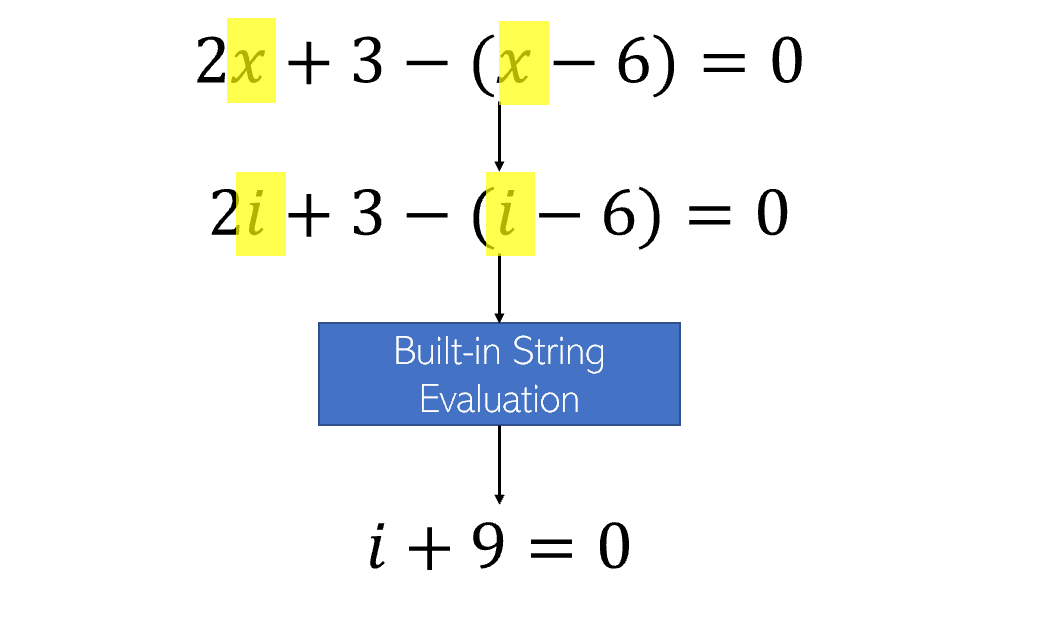python linear equation 5

First, as a demonstration of how eval() works, in that it can evaluate any Python command given in a string:

``````>>> eval("print('This is output')")
This is output
``````

Hence, mathematical expressions are expressed like any Python expression would be processed. The genius of using eval() is that instead of manually doing the heavy lifting, we utilize Python’s string processing methods.

The string is evaluated automatically by tricking Python into believing that x is actually i.

Note that this is the same as…

Finally, we have achieved a simplified form ax + b = 0. Through standard and simple algebra manipulation, we find that x is equal to -b/a, or, in relation to the complex number we created, the negative of the real component (9 in the example above) divided by the imaginary component multiplier (1 in the example above).

``````return -grouped.real/grouped.imag
``````

The last line of code simply returns this algebraic step by returning the negative of the real component of the complex number divided by the imaginary component.

This three-line function works on any linear equation, regardless of length or complexity, by manipulating and harnessing the power of Python’s built-in math evaluation.

If you enjoyed, feel free to upvote!

# SUBSCRIBE FOR NEW ARTICLES